Strong shape theory

A strong shape category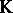has topological spaces with, or without, base points, respectively, as objects and strong shape mappingsas morphisms (cf. also Category; Topological space). There are strong shape categories for arbitrary topological spaces, for compact metric spaces and (stably) for separable metric, finite-dimensional spaces (the compact-open strong shape morphisms are called-morphisms). The main objective of strong shape theory is to perform algebraic topology (i.e. generalized homology and cohomology theory, (stable) homotopy theory,-duality and eventually fibre theory) for more exotic spaces in the same way as one did in ordinary homotopy theory for CW-spaces (cf. also Cohomology; Cohomology of a complex; CW-complex).

Let, for example,be a spectrum (cf. also Spectrum of spaces) anda CW-space. Then the generalized (co)homology ofwith coefficients in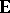is defined by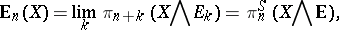respectively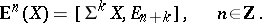Suppose one is working with compact metric spacesand replaces, in the previous definition of homology, continuous mappings by strong shape mappings. Then one obtains again a homology theory,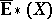, the Steenrod–Sitnikov homology ofwith coefficients in.

For even more general spaces, a kind of homology theory which lives in a strong shape category is the so-called strong homology. It can be characterized by a set of axioms very similar to the Milnor axioms for Steenrod–Sitnikov homology (the Steenrod–Eilenberg axioms without a dimension axiom, but with a very strong excision axiom and a kind of continuity axiom (on a chain level), resembling Milnor's cluster axiom).

Steenrod–Sitnikov homology is the appropriate tool for establishing Alexander duality theorems [a2] (cf. also Alexander duality):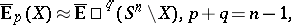(a1)

for suitable (e.g. CW-) spectraand spaces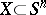.

The corresponding cohomology reveals itself as a Čech-type cohomology, cf. also Čech cohomology.-duality for arbitrary separable metric, finite-dimensional spaces,guarantees the existence of a duality functorsatisfying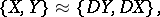(a2)Here,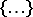on both sides of (a2) are in fact-morphisms between objects in an-category [a3]. The associated result for ordinary shape and compactgoes back to E. Lima [a8], while there is a corresponding theorem for compacta and strong shape by Q. Haxhibeqiri and S. Novak [a7]; both of them are, unlike (a2), not symmetrical, because the-dual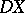of a compact space need no longer be compact.

Since, unlike for ordinary shape, one has in a strong shape category access to individual shape morphisms (rather than merely to their homotopy classes), one can define a shape singular complex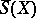, a Kan-complex, which is achieved in the same way as the singular complexof a space, but with individual strong shape morphisms replacing continuous mappings.

In particular, this allows the construction of a kind of shape singular homology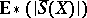, which turns out to be the appropriate generalization of Borel–Moore homology.

Strong shape theory has been independently discovered by A.D. Edwards and H.M. Hastings [a5] and F.W. Bauer [a1]. More recently, B. Guenther [a6] introduced an ultimate model for a strong shape category for arbitrary topological spaces. Subsequently, numerous authors have contributed to strong shape theory, like S. Mardešić (notably his theory of ANR expansions of a space, cf. [a6] for further references or Retract of a topological space), J. Segal, Y. Kodama ( "fine shape" ) and others.

An ordinary shape mapping is a "machine" (in fact, of course, a functor between appropriate categories), assigning to a homotopy class, witha "good space" (e.g. a polyhedron, a CW-space or an absolute neighbourhood retract), a homotopy class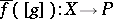, respecting homotopy commutative diagrams (i.e. if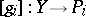,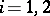andare given such that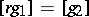, then the associated diagram foris homotopy commutative (cf. also Diagram).

In strong shape theory one has to work with individual mappings (rather than with homotopy classes) and with specific homotopies: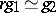instead of the more unspecific statement thatis homotopic to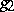. Since this involves higher homotopies of arbitrary high degree, one has to deal with-categories and-functors for arbitrary high.

This is very similar to the approach taken by K. Sitnikov to (ordinary) Steenrod–Sitnikov homology, where one has (other than for Vietoris or Čech homology) to replace homology classes by individual cycles and homology relations by specifying individual connecting chains between cycles.

The strong shape homotopy category for compacta turns out to be equivalent to a quotient category of the category of compacta (with continuous mappings as morphisms) by converting all so-called SSDR-mappings into equivalences [a4]. Ordinary shape does not have this property.